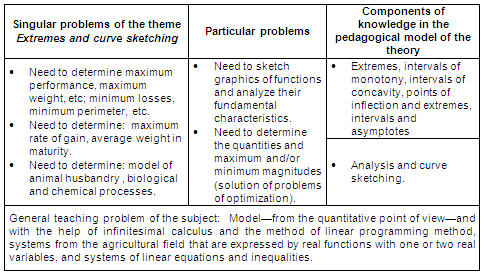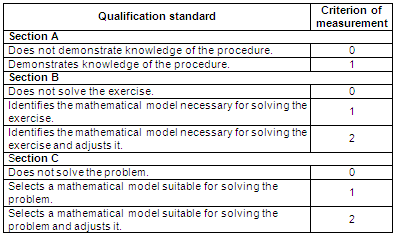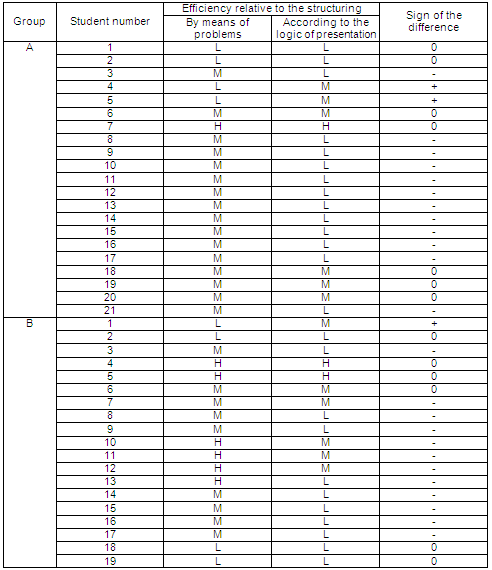Revista Electrónica de Investigación Educativa

Vol. 6, No. 2, 2004

Structuring Mathematical Context by Means
of Problems: A Mechanism for Achieving
Effective Knowledge in Higher Education

Eloy Guerrero Seide
chavez@infosol.gtm.sld.cu

Departamento de Matemática y Computación

Calle Sol 770
Zona postal 95100
Guantánamo, Cuba

(Received: December 12, 2003; accepted for publishing: June 14, 2007)

Abstract

This article summarizes the results obtained in an exploratory and comparative study of two ways of structuring the mathematical content of a B.S. program in Agronomic Engineering at Guantanamo University, Cuba: the formal systematization of the presentation of the knowledge, and an organization through problems. The sign test is used in the proof of the hypothesis. In a preliminary form, at least, it was demonstrated that the variant of systemic structuring of knowledge through problems is more conducive to the efficiency of the knowledge acquired by students than the structure presented by means of the logical exposition of achieved knowledge.

Key words: Mathematics teaching, problem-based learning.

Introduction

Based on the presuppositions of cognitive theories and their application in the teaching-learning process, this paper presents the results of a study which explores the relationship between the structure of the content of a curriculum of Mathematics (in the B.S. Program of Agronomic Engineering of Guantanamo University, Cuba) and the efficiency of the knowledge obtained by students. The dependent and independent variables determined were: the efficiency of knowledge and knowledge structure in the program, respectively.

For the independent variable, two states are considered, that is, two modes of structuring the content: the first, by means of problems, and the second, by following the procedure of structuring the formalization of mathematical knowledge already attained.

The characteristic efficiency of knowledge is quantified by means of a scale with three categories or levels: low, medium, and high.

The term efficiency of mathematical knowledge (relative to a specific program) of a student was assumed in the study as the development of skills and habits necessary to recognize, in a given situation the conditions suitable for the use of a specific mathematical model, adapt this model, develop the formal calculus procedures to find the “quantitative solution”, and finally, to make the appropriate interpretation of the solutions found.

The study was conducted by comparing the effects of both structures on the efficiency of the knowledge acquired by the students. The scientific work was oriented to answer, at least in preliminary form, the question, “Is there a difference in the efficiency of the mathematical knowledge obtained by students when they are submitted to an organization of knowledge following the logic of formal presentation, and another, alternative organization of knowledge through problems?”

To answer the scientific question, an experiment was conducted with two first-year groups in the study program mentioned, in the Agroforestry School of the University of Guantanamo, during the second semester of the 2001-2002 school year. The experiment consisted of two stages, which were structured corresponding with the two selected variants. At the end of each period, measurements of the students’ efficiency were made.

The results of the experiment contributed elements that approximated a demonstration of the hypothesis: the organization by problems contributes to the students’ acquiring efficient mathematical knowledge for their professional development.

The object of the study was to prove that in the work of teaching the subject of mathematics, the efficiency of the students’ acquired knowledge is greater when the content of the program is structured through problems, than when it is structured through the logical presentation of achieved mathematical knowledge.

The hypothesis

a) Null hypothesis

The structure of knowledge in the program of the course Mathematics II, under the logic of the formal presentation of knowledge and by means of problems, produces the same effects on the efficiency of knowledge acquired by students.

b) Alternative hypothesis

The structure of knowledge by means of problems in the program of the course Mathematics II has a greater influence on the level of efficiency students reach, than the structure following the logic of presentation.

Method

The experiment was conducted with all students enrolled in the two first-year groups of the 2001-2002 Agronomical Engineering degree program at the Agroforestry School of Guantanamo University (CUG). At the time of the experiment, the enrollment of the groups was 21 and 19 students respectively. The 40 students were living in the university’s dormitories, with similar alimentary conditions, access to scientific/technical information (physical support, Internet and other media) and having material and financial insurance. All the students were from rural zones with similar socioeconomic conditions.

The subject selected was Mathematics II, which is part of the mathematics discipline.

The experiment was conducted during the February-April quarter of 2002, and lasted eight weeks. However, none of the students knew that the experiment was going on.

During the first of the two stages of the experiment, the content used was Extremes (of real functions with one and two variables) and curve tracing, organized through the use of problems.

For the second stage, the content selected was Ordinary differential Equations (ODE) of the first order and second order linear ODEs with constant coefficients, homogeneous and inhomogeneous.

It should be noted that in the experiment each group was compared with itself at the time of the analysis of the measurements. The irreversibility of learning causes the efficiency of the knowledge obtained with the second structure to be influenced by the efficiency of the knowledge derived from the work with the first. From this it follows that the efficiency levels produced in students by work with the ODE (content structured with the logic of presentation of mathematical knowledge, as results) were affected by the efficiency of the learning produced during the work with applications of differential calculus (extremes and sketching plane curves; content structured by means of problems).

For purposes of the test, the sequence chosen for the work with the structures is unfavorable, given that it creates conditions so that the null hypothesis is completed instead of being rejected. Then, in this case, the rejection of the null hypothesis augments the credibility of the result: “accept the alternative hypothesis”.

The groups were taught by the same teacher, and the independent variable was the structuring of the system of knowledge in the program of the subject.

The dependent variable selected was the efficiency of the knowledge obtained by the students.

Independent variable: didactic procedure of structuring the knowledge system by means of problems

In the experiment, two variants of the systemic structuring of knowledge were considered. These were obtained by the implementation of procedures for structuring: one, following the logic of the presentation of knowledge already obtained, which mathematical science uses; and the other, through the use of problems.

The procedure by using problems, as it has been conceived, is applicable to any of the organizational levels of the teaching process of a study program (a discipline, a subject or a topic).

The process of structuring the knowledge system and the system by means of problems generated by this procedure have been called, respectively: didactic procedure of the property of solubility, and solubility relations system.

The didactic procedure of systemic structuring of knowledge (Guerrero, 2001) is based on a dialectical concept of systems. In this focus, the study of the system is carried out by making known the beginning (the initial, elemental form of everything) and the laws that determine the genesis and the development of the system.

In the procedure being analyzed, the beginning is given by the kind of problems generated (generative network) of the knowledge programmed. These problems express the need for new knowledge, and in turn, are carriers of the relationships (of solubility) between the theoretical/mathematical knowledge of study in a subject and its applications. In other words, by means of the kind of “generative” problems there are revealed: the relationships between the knowledge in use and the demands of the practice that require new mathematical knowledge. Which could justify the term “solubility relationships”.

From the logical-epistemological standpoint in any of the three organizational levels of the teaching process of a study program: discipline, subject or topic, the didactic procedure of the property of solubility has as support the understanding that one should begin based on needs, as an essential and unavoidable condition for explaining the acts of man. So affirms Frederick Engels (1982) in his work Dialectics of Nature.

If we accept that cognitive problems express needs to find new knowledge, then problems represent fit starting points suitable for explaining the emergence of knowledge in the teaching process, with which the learning of such knowledge and that of the relationships which exist between the problems and the knowledge associated with them is potentiated, in the sense of the latter’s serving to solve the former.

It seems clear that the procedure of the property of solubility as teaching strategy for structuring the system of knowledge of a mathematics course, presupposes in an implicit or explicit manner a "non a priori" concept of the origin of the objects of the knowledge.

The connections of mathematical knowledge with the economic and social development of mankind can produce effects of motivation for the study of the subject, above all if they are suitably selected within the class of causative problems, those linked with the profession in whose course of study the subject is inserted (or the discipline). Marie Lise Peltier (1993) in an analysis of the trajectory of mathematics teaching in France expressed the advisability (for its educational function) of combining “learning situations” with the dual character of mathematical knowledge: an instrument (in solving a problem) and an object (of knowledge). In this sense, Peltier argued that knowledge first plays the role of an instrument in the solving of a problem, and that only then can it become the object of knowing.

In the generative network, problems are distributed in classes serving the level of generality with which the needs of the new knowledge are expressed. Depending of their degree of generality, they can be: singular problems expressed in the form of concrete tasks, particular problems that summarize as a generalization the common features of the singular problems of a class, and finally, the so-called general problems which are generalizations of groups of particular problems.

The concepts and procedures incorporated as new tools for solving a particular problem are established with the requirement of invariance, which means independence in relation to singular subjects who use them in solving the task; also in respect to the specific properties of the object of each of the singular problems that form part of the class.

The analysis which leads to abstraction and especially to the extensive use of analogy and idealization makes it possible to establish the particular problem associated with each family or class of singular problems. Each one is a generalization of the essential cognitive needs, expressed in a family of singular problems (Guerrero, 2001).

The method of solving a particular problem is suitable for solving any of the singular problems that underlie their respective class, although the precise method needs certain adjustments for the concrete cases.

The triad knowledge in use-new cognitive needs-new knowledge that marks in a general sense the path of knowledge development, has its expression in the genesis and development of the system of contents programmed in the learning process. The subsystems of particular problems are carriers of the contradictions between the thesis (knowledge in use) and the antithesis (the new needs). For its part, the synthesis is given by the theories that systematize the new knowledge.

Table I summarizes the singular problems and the particular problems expressed, and the components used from the didactic model of the theory, in the implementation of this concept of systemic structure of knowledge in the topic Extremes and curve sketching, selected from the program of Mathematics II.

Table I. Summary of a network of problems for the subject Extremes of functions (of one and two variables) and curve sketchingDependent Variable: Efficiency of mathematical knowledge attained by students

The efficiency of mathematical knowledge is defined as the necessary unit the student establishes between knowledge, skills and specific habits of the subject, as well as the general scholastic skills and habits, to apply them in solving concrete problems, which presupposes transforming or adapting the procedures studied to the resolution of new situations.

To evaluate the efficiency of knowledge in each of the stages of the experiment there was applied a pedagogical test, which had three sections designated A, B, and C. In Section A were included formal exercises, that is, those whose statements indicated the mathematical operations to be performed to find the solution. In Section B were expressed exercises with texts, in which appeared a “key word” that enabled the student to recognize the mathematical model for solution. The third and last section, Section C, contained exercises in the form of problems, for whose solution the student had to decide by means of the stated situation, which one(s) of the mathematical models s/he should use. In solving the exercises in Section C, the student was also required to adapt the model to the specific circumstances described; and finally, to solve the problem, and interpret the solution.

The efficiency measurement was performed according to the qualification standards presented in Table II.

Table II. Qualification standards for the three sections of the pedagogical
test applied to evaluate the efficiency of knowledge
at each stage of the experimentThe final score for the quantification of the efficiency of knowledge was calculated using an additive scale, which was weighted with a total of 2 points for section C.

Finally, to measure the efficiency of the knowledge students attained at each stage, the following categories were considered: (A) 6 - 7, high, (M) 3 - 5, medium, (B) 0 - 2, low.

Results

Efficiency levels of knowledge acquired by students under the influence of the respective structures of knowledge (with the logic of presentation and by means of problems) in the program of the course Mathematics II are shown in Table III.

Table III. Levels of knowledge efficiency achieved by students in both types of structuring (L = 0 to 2, low level; M = 3 to 5, medium level; H = 6 to 7, high level)Using the sign test, we found that there were 23 minus signs, 3 plus signs, and 14 cases in which there was no change.

In the null hypothesis it has been assumed that the probability of obtaining a minus sign is p = 0.05; in contrast, in the unilateral alternative hypothesis it is supposed that the probability is p> 0.50. As we have analyzed, there were obtained 23 successes in 26 tests. In proceeding to approximate the normal curve to the binomial distribution, we obtained z = 3.92, and since it exceeds the critical value of 2.33 (for a unilateral test with significance level 0.01) the null hypothesis could be rejected, and the alternative accepted.

Conclusions

The experience developed offers elements which suggest that the variant of systemic structuring of knowledge by means of problems in the B.S. Program in Agronomic Engineering at Guantanamo University has greater effects on the efficiency of knowledge acquired by students, than the systemic structure of knowledge expressed by means of the logic of the presentation of knowledge achieved, commonly used in mathematical science.

The structuring of mathematical knowledge in training programs for professionals, following the logic of the presentation of knowledge already achieved, reflects only one of the two segments of the real path of knowledge, since the presentation of the knowledge system by means of the systematic presentation of mathematical science omits the analysis of the genesis and development of new knowledge; and ignores, in many cases, the need for the emergence of the knowledge and resources of solubility contributed by theories in the processes of their application in solving the types of problems.

As for the structure of mathematical knowledge through problems in the program of the course Mathematics II of the study program Agronomic Engineering, it models the hypothetic-deductive logic and promotes professional activity in the solution of the quantitative aspects of the problematic situations that present themselves in the relevant field of action.

References

Engels, F. (1982). Dialéctica de la naturaleza. La Habana: Editorial de Ciencias Sociales.

Guerrero, E. (2001). Una variante para la estructuración del contenido de la disciplina. Análisis Matemático de la carrera Matemática Computación en los institutos superiores pedagógicos. Unpublished doctoral dissertation. Universidad de Guantánamo, Guantánamo, Cuba.

Peltier, M. L. (1993). Una visión general de la didáctica de las matemáticas en Francia. Revista Educación Matemática, 5 (2), 4-10.

Translator: Lessie Evona York Weatherman
UABC Mexicali

1In Cuba the design of the curriculum is produced on the basis of the dichotomy centralization-decentralization, which envisages the centralized production of the principles that govern said plans, and the adaptation of such plans by each institution of higher education to its own specific conditions. In this order, in plans now in force there rules the so-called curriculum model of conscious processes, in which the following are considered as organizational levels of the learning process: the university course of study, discipline, subjects and themes. Each study program has a number of disciplines; these bring together subjects which are in turn made up of a specific number of themes.

2Accepting the objectivity of the origin of mathematical knowledge does not mean conceiving an origin in material objects for them in every case; on the contrary, it is accepted that in many cases knowledge of this type has another type of origin.